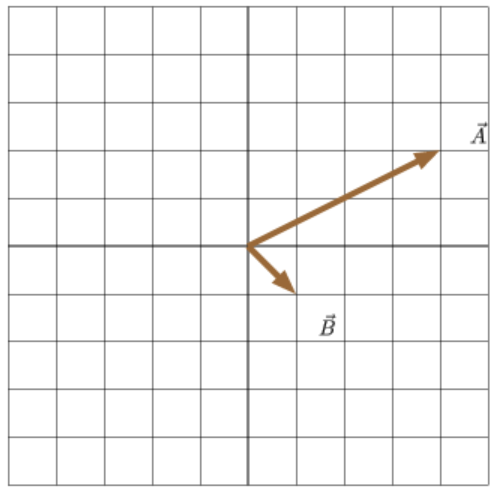# Problem: Draw the vector C→=0.5A→-2B→. The length and orientation of the vector will be graded. The location of the vector is not important.

###### FREE Expert Solution

$\stackrel{\mathbf{⇀}}{\mathbf{A}}\mathbf{=}\mathbf{4}\stackrel{\mathbf{^}}{\mathbf{i}}\mathbf{+}\mathbf{2}\stackrel{\mathbf{^}}{\mathbf{j}}$

$\mathbf{0}\mathbf{.}\mathbf{5}\stackrel{\mathbf{⇀}}{\mathbf{A}}\mathbf{=}\mathbf{2}\stackrel{\mathbf{^}}{\mathbf{i}}\mathbf{+}\mathbf{1}\stackrel{\mathbf{^}}{\mathbf{j}}$###### Problem Details

Draw the vector $\stackrel{\mathbf{\to }}{\mathbf{C}}\mathbf{=}\mathbf{0}\mathbf{.}\mathbf{5}\stackrel{\mathbf{\to }}{\mathbf{A}}\mathbf{-}\mathbf{2}\stackrel{\mathbf{\to }}{\mathbf{B}}$

The length and orientation of the vector will be graded. The location of the vector is not important.